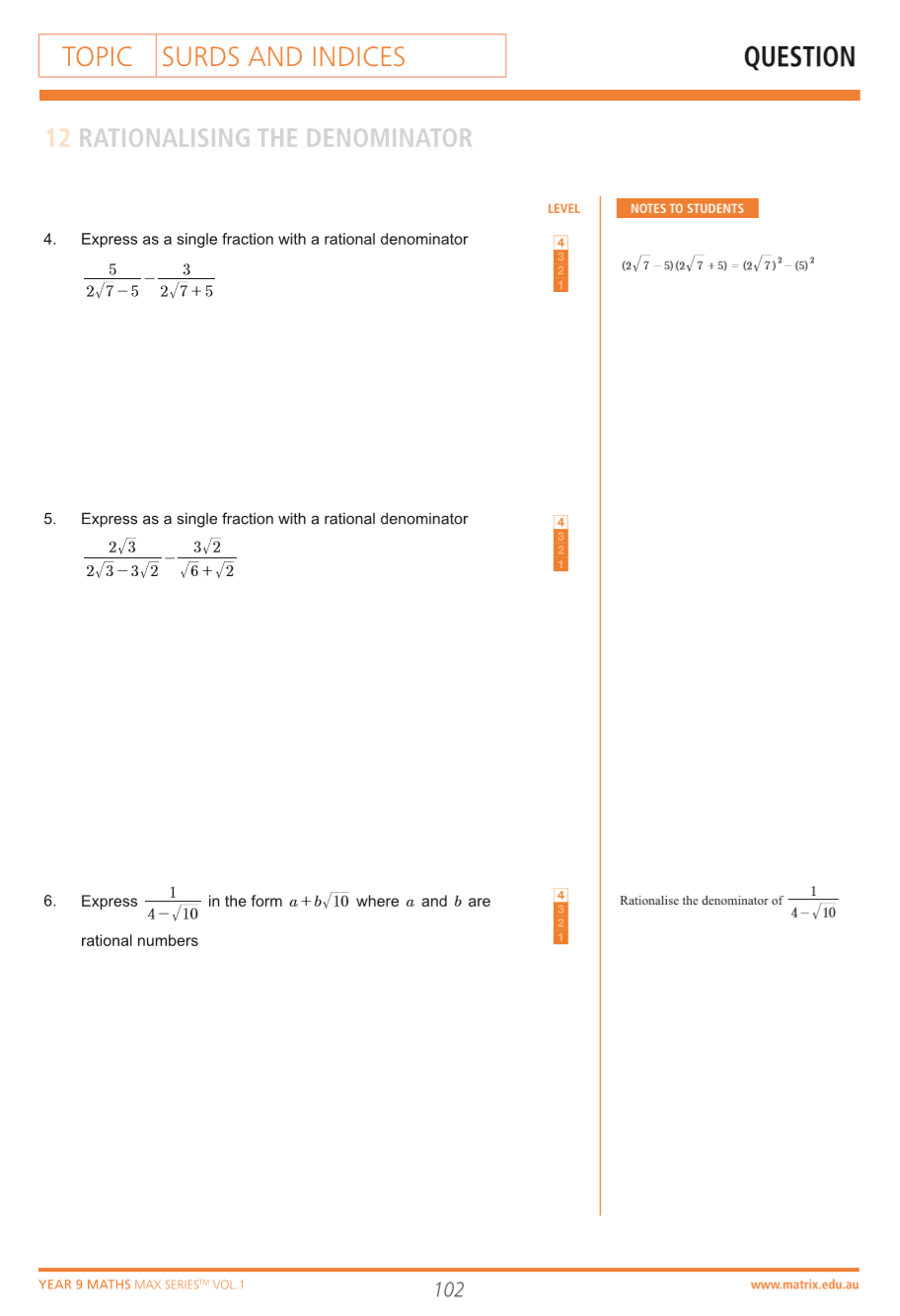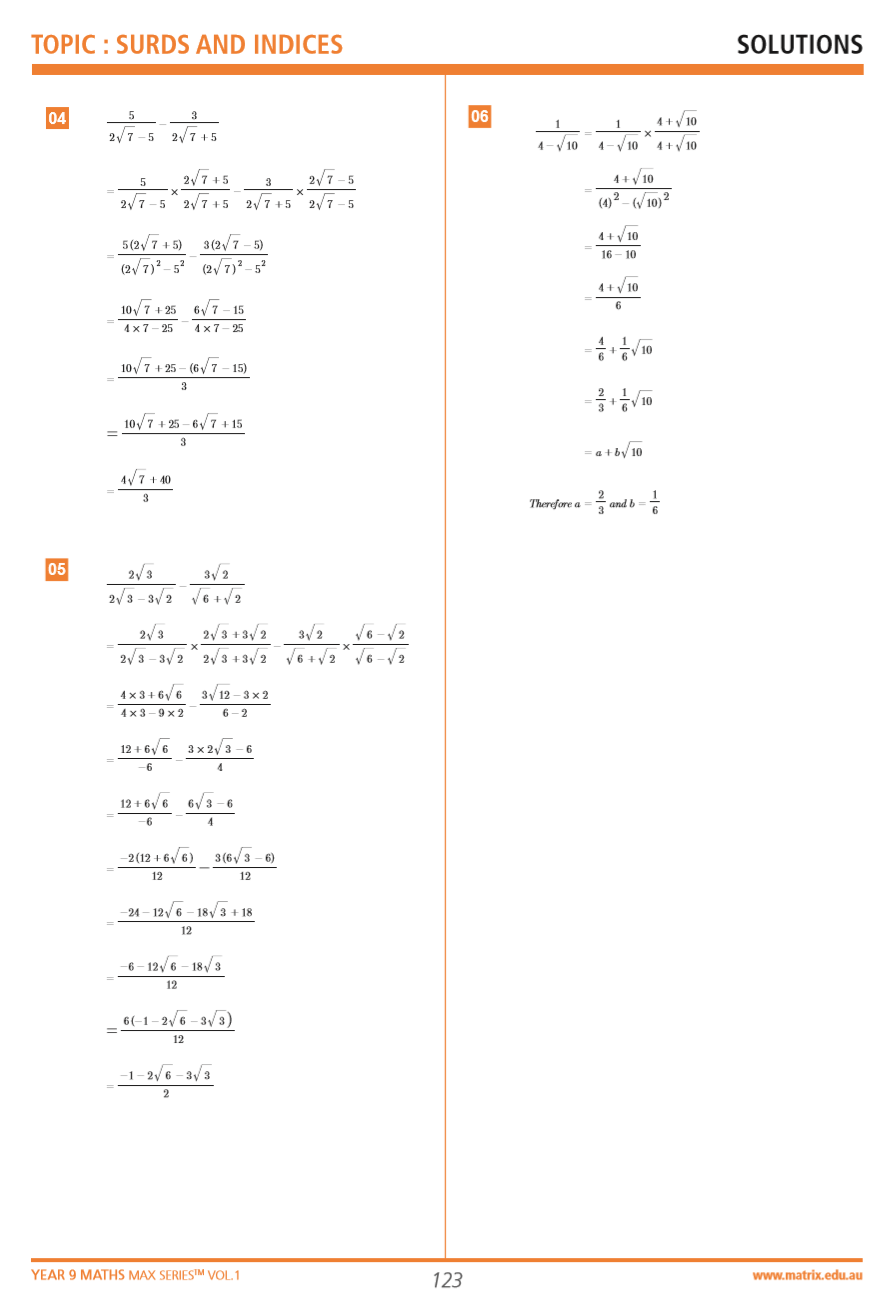# Part 4: Surds | Free Worksheet

In this article, we'll show you the skills you need to subdue your surds anxiety.## Surds

Are you struggling with surds? You’re not alone. But understanding surds is an essential foundation for later stages of maths. So, we’re going to give you a comprehensive guide to help you conquer them.

## Syllabus Outcome

This article addresses the following syllabus outcomes:

Surds and Indices:

• Uses and interprets formal definitions and generalisations when explaining solutions and/or conjectures. MA5.3-1WM
• Generalises mathematical ideas and techniques to analyse and solve problems efficiently. MA5.3-2WM
• Uses deductive reasoning in presenting arguments and formal proofs. MA5.3-3WM
• Performs operations with surds and indices. MA5.3-6NA

This may seem like a long and convoluted set of statements. But what it is saying is really quite straightforward.

Essentially, these Outcomes state that in this unit you will learn that we have index laws for numbers and we have to adhere to these laws to solve problems.

## In this article we discuss

This topic introduces you to operations involving surds.

You are expected to evaluate more complex expressions involving negative, zero and fractional indices, including simplification and binomial products.

Often, students have trouble simplifying surds and using index laws correctly. They tend to become confused and forget the fundamentals of the indices and surds topic when they get overwhelmed with a combination of different techniques.

Common mistakes include:

• Uncertainty around the role of brackets in expressions
• Incorrect simplification of more complex expressions

Manipulating surds and indices can be challenging, especially when you have to combine multiple ideas from both topics to get the correct answer – however, not to worry!

This subject guide will point out common errors and help you better understand these new concepts.

## Assumed knowledge

There is some prior knowledge needed before starting this topic. You should have a basic understanding of surds.

For example, the root function – what is $$\sqrt{3}$$? How do you find the square root of a number?

You’ll also need to be across indices.

For example, what does $$2^{3}$$ mean? What does $$3^{2}$$ mean? How are they different?

Additionally, you should be familiar with elementary BODMAS (Bracket, Of, Division, Multiplication, Addition and Subtraction) operations and simple algebraic expressions.

If you aren’t already comfortable with the concepts above, it’s a good idea to revise them before starting this topic – it will be extremely useful.

Take the time to read the previous article on indices, and test yourself on the worksheet, if you haven’t already.

## See how well you know Surds## Surds

As we’ve explored in the definitions in the previous post, most surds are irrational numbers and cannot be written as fractions. There are special ways of treating surds in operations.

Let’s look at what they are:

## Multiplication:

### Rules:

$$\sqrt{a} × \sqrt{b}= \sqrt{ab} \\ \sqrt{a^2}=(\sqrt{a})^{2}=a \\ a\sqrt{b} × c\sqrt{d}= ac\sqrt{bd}\\$$

When multiplying two surds, you first multiply the values under them, then put it under the same root.

$$\sqrt{3} × \sqrt{5}= \sqrt{3 × 5}=\sqrt{15}$$

When multiplying surds that have an integer outside of it, multiply the integers and surds separately.

$$5\sqrt{2} × 3\sqrt{7}= 15\sqrt{14}$$

### Checkpoint questions:

1. $$\sqrt{3} × \sqrt{12}$$

2. $$2\sqrt{5} × \sqrt{2}$$

3. $$(4\sqrt{3})^{3}$$

### Answers:

Using the first rule, we multiply the two values under the surds first:

1. $$\sqrt{3×12}=\sqrt{36}$$, and using the second rule, $$\sqrt{36}=\sqrt{6^{2}}=6$$

2.Using the third rule, the integers and surds are multiplied separately to get $$2\sqrt{10}$$

3.This can be treated as $$(4\sqrt{3}) × (4\sqrt{3}) × (4\sqrt{3})$$, which equals $$64 \sqrt{27}$$.

## Simplification:

For many of these operations, you will have to simplify the surd first. A surd is in its simplest form when the number under the square root sign is not a factor of a perfect square.

For example, $$\sqrt{8}$$ is not a simplified surd as $$4 = 2^2$$ is a factor of 8. However, $$\sqrt{10}$$, is a simplified surd as it does not have any factors that are perfect squares.

Students often have trouble understanding this.

Here is a breakdown of surd simplification. Let’s work with the example $$\sqrt{128}$$.

### Step 1

Look for a square number that is a factor of the integer inside the root. If you can’t spot one immediately, start with small numbers such as $$4$$ or $$9$$ – the simplification process can be done multiple times after this.

For $$\sqrt{128}$$, we can see that $$128$$ is divisible by $$64$$ – a perfect square!

### Step 2

Write the inside of the surd as the product of the perfect square and its other factor.

$$\sqrt{128} = \sqrt{64×2}$$

### Step 3

Using Rule 1, we know that the reverse is true as well. Hence we are able to split the number into two different surds.

$$\sqrt{128}= \sqrt{64×2}= \sqrt{64}×\sqrt{2}$$

### Step 4

Here is where the perfect square is used. We know that the root of a perfect square simplifies down to an integer. Therefore, we can just eliminate the square root and multiply the resulting integer with our other root.

$$\sqrt{64} × \sqrt{2}=8×\sqrt{2} =8\sqrt{2}$$

It is useful to become familiar with this process – it allows you to deal with a much wider range of surds.

## Division:

When dividing two surds, you divide the values inside them first, then put them under the same root.

$$\sqrt{\frac{a}{b}}=\frac{\sqrt{a}}{\sqrt{b}}$$ $$\frac{a\sqrt{b}}{c\sqrt{d}}=\frac{a}{c}×\sqrt{\frac{b}{d}}$$

Have a look at the following:

1. $$\sqrt{\frac{24}{8}}$$

The fraction inside the surd can be simplified first to $$3$$. The answer is then just $$\sqrt{3}$$.

2. $$\frac{3\sqrt{8}}{\sqrt{2}}$$

At first glance, it seems that we aren’t able to solve this. But – remember we are able to simplify! Using what we know, $$\sqrt{8}$$ can be written as $$2\sqrt{2}$$.

Then the fraction becomes $$\frac{3×2\sqrt{2}}{\sqrt{2}}$$. We can cancel out the $$\sqrt{2}$$ to give $$6$$.

Using the same method as above, simplify:

$$\frac{\sqrt{99}}{4\sqrt{3}}$$.

## Addition and Subtraction:

You’ve been introduced to how addition and subtraction of algebraic terms works; a similar idea applies to addition and subtraction of surds. Like $$x$$’s and $$y$$’s, surds can only be added or subtracted from each other if they are ‘like’ – that is, if they have the same value under the surd.

$$\sqrt{3}+\sqrt{5}$$ is NOT equal to $$8$$, as $$3$$ and $$5$$ are not “like” terms – they are completely different surds.

$$2\sqrt{3}$$ essentially means there are “$$2$$ of $$\sqrt{3}$$‘s” – in other words $$\sqrt{3}+\sqrt{3}$$.

That’s why, if you were to evaluate $$2\sqrt{3}+ \sqrt{3}$$, it would be $$3\sqrt{3}$$; this is similar to saying $$2x+x=3x$$

Similarly, $$6\sqrt{5}+2\sqrt{5}=8\sqrt{5}$$ (think: $$6x+2x=8x$$)

A similar concept applies to subtraction; only “like” terms can be subtracted.

### Question:

What is $$8\sqrt{5}-12\sqrt{5}-7\sqrt{5}+ 3\sqrt{10}$$?

Here we see that there are two different terms; $$\sqrt{5}$$ and $$\sqrt{10}$$, so these have to be treated separately to each other. We can think of the expression as something like $$8x-12x-7x+3y$$ – how would you solve this?

Now solve the above in the same way – it just becomes $$-11\sqrt{5}+3\sqrt{10}$$.

## Rationalising the denominator

Recall our definition of a rational number – it is one that can be written as a fraction. Now, when we have a denominator in the form of a root (eg. $$\sqrt{a}$$, where a is a rational number, and hence irrational), we want to make it a rational number (hence the term ‘rationalise’).

Think about what we can multiply $$\sqrt{a}$$ by to make it rational: $$\sqrt{a}$$ itself! Doing this turns it into $$a$$, which we know is a rational number.

Hence, to rationalise a single term denominator of a fraction, simply multiply both numerator and denominator by the surd.

### For example:

Rationalise the denominator for $$\frac{2}{\sqrt{3}}$$

### Step 1:

Multiply both numerator and denominator by the surd in the denominator

$$\frac{6}{\sqrt{3}} ×\frac{\sqrt{3}}{\sqrt{3}}$$

Since $$\frac{\sqrt{3}}{\sqrt{3}}=1$$, we’re not changing the fraction in any way; we are essentially just multiplying it by $$1$$.

### Step 2:

Simplify the fraction if possible.

$$\frac{6}{\sqrt{3}} ×\frac{\sqrt{3}}{\sqrt{3}}=\frac{6\sqrt{3}}{3}$$

The $$6$$ in the numerator and $$3$$ in the denominator can cancel out to give our result, $$2\sqrt{3}$$.

Another example:

$$\frac{2\sqrt{5}-3\sqrt{2}}{\sqrt{5}}$$ $$= \frac{\sqrt{5} (2\sqrt{5}-3\sqrt{2})}{\sqrt{5}×\sqrt{5}}$$ $$=\frac{10-3\sqrt{10}}{5}$$

## Surds:

### Multiplication

$$\sqrt{a} × \sqrt{b}= \sqrt{ab}$$
$$\sqrt{a^{2}}=(\sqrt{a})^{2}=a$$
$$a\sqrt{b} × c\sqrt{d} = ac\sqrt{bd}$$

### Division

$$\frac{a\sqrt{b}}{c\sqrt{d}}=\frac{a}{c}×\frac{b}{d}$$

### Simplification

$$\sqrt{k^{2} × a}=k\sqrt{a}$$

### Rationalisation

$$\frac {\sqrt{b}}{\sqrt{a}}=\frac{\sqrt{b}×\sqrt{a}}{\sqrt{a}×\sqrt{a}}=\frac{\sqrt{ab}}{a}$$

## What should you do with these?

You should be able to remember all of these. This sounds daunting. However, with enough practice, you will be able to know them off by heart and be readily able to apply them in your calculations. Just practise by doing as many questions as you can!

If you encounter a question that looks especially complex and difficult, remember to recall your basic understanding of the surds and indices rules and tackle the question step-by-step.

If you are able to break down the question into chunks, it’s a lot easier to think clearly and to manage each chunk individually. It’ll eventually boil down to a simple expression!

Take note of the common mistakes that students make and remember this while doing questions.

## Additional Challenging Examples (From the MAX Series)

### QuestionsQuestions from Year 9 Maths Max Series Vol. 1 Surds and Indices

### AnswersAnswers from Year 9 Maths Max Series Vol. 1 Surds and Indices

## Need more practice?

With our Term Course for Year 9 Maths, you will revise over core concepts and apply your skills to succeed in Maths!

© Matrix Education and www.matrix.edu.au, 2021. Unauthorised use and/or duplication of this material without express and written permission from this site’s author and/or owner is strictly prohibited. Excerpts and links may be used, provided that full and clear credit is given to Matrix Education and www.matrix.edu.au with appropriate and specific direction to the original content.

### Get free study tips and resources delivered to your inbox.

Join 75,893 students who already have a head start.

Our website uses cookies to provide you with a better browsing experience. If you continue to use this site, you consent to our use of cookies. Read our cookies statement.